Maths-
General
Easy

Question

# If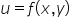is a homogeneous function of order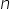.I)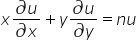II)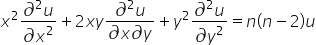III)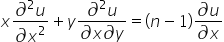Which of the above statements is correct ?

## Only IOnly II, IIIOnly I and IIII, II, IIIHint:

## The correct answer is: Only I and III

### We are given that u is a homogeneous function of degree  n and it is function of x and y. We will check all three statements one by one.I. For first statement, we will take the partial derivative of u w.r.t x and y. We are using Euler's theorem to write the derivative.Euler's theorem states that if u is homogeneous function of x and y having degree n, then we can write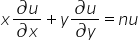...(1)The first statement is correct.II. For second statement, we need second order derivative. We will take the partial derivative of equation (1) w.r.t x and then y.Take partial derivative w.r.t xMultiply the equation (2) by x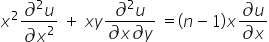...(3)Similarly, take the partial derivative of equation (1) w.r.t y.Multiply equation (4) by y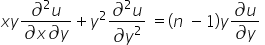...(5)Adding (4) and (5) we get,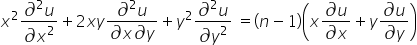From (1) we get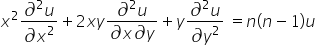So, statement "II" is incorrect.III. See equation no (2).We can say that statement III is correct.So, the option which says "only I and III", is the right option.

We have to remember all the three results instead of deriving them.#### With Turito Foundation.#### Get an Expert Advice From Turito.